# Find the smallest number that must be subtracted from those of the numbers in question 2 which are not perfect cubes,`
Question:

Find the smallest number that must be subtracted from those of the numbers in question 2 which are not perfect cubes, to make them perfect cubes. What are the corresponding cube roots?

Solution:

(i)
We have: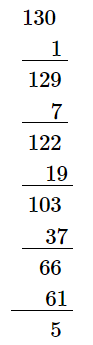$\because$ The next number to be subtracted is 91 , which is greater than 5 .

$\therefore 130$ is not a perfect cube.

However, if we subtract 5 from 130, we will get 0 on performing successive subtraction and the number will become a perfect cube.

If we subtract 5 from 130, we get 125. Now, find the cube root using successive subtraction.

We have: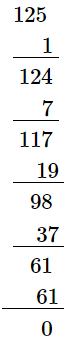$\because$ The subtraction is performed 5 times.

$\therefore \sqrt{125}=5$

Thus, it is a perfect cube.

(ii)
We have: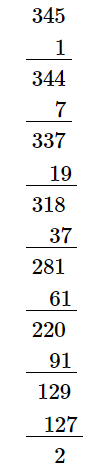$\because$ The next number to be subtracted is 161 , which is greater than 2 .

$\therefore 345$ is not a perfect cube.

However, if we subtract 2 from 345, we will get 0 on performing successive subtraction and the number will become a perfect cube.

If we subtract 2 from 345, we get 343. Now, find the cube root using successive subtraction.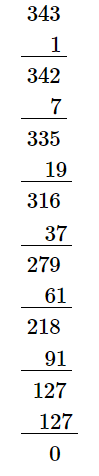$\because$ The subtraction is performed 7 times.

$\therefore \sqrt{343}=7$

Thus, it is a perfect cube.

(iii)
We have: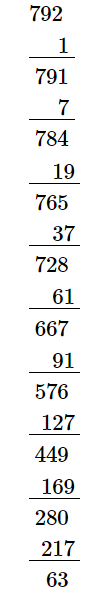$\because$ The next number to be subtracted is 271 , which is greater than 63 .

$\therefore 792$ is not a perfect cube.

However, if we subtract 63 from 792, we will get 0 on performing successive subtraction and the number will become a perfect cube.

If we subtract 63 from 792, we get 729. Now, find the cube root using the successive subtraction.

We have: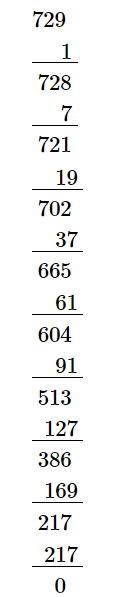$\because$ The subtraction is performed 9 times.

$\therefore \sqrt{729}=9$

Thus, it is perfect cube.